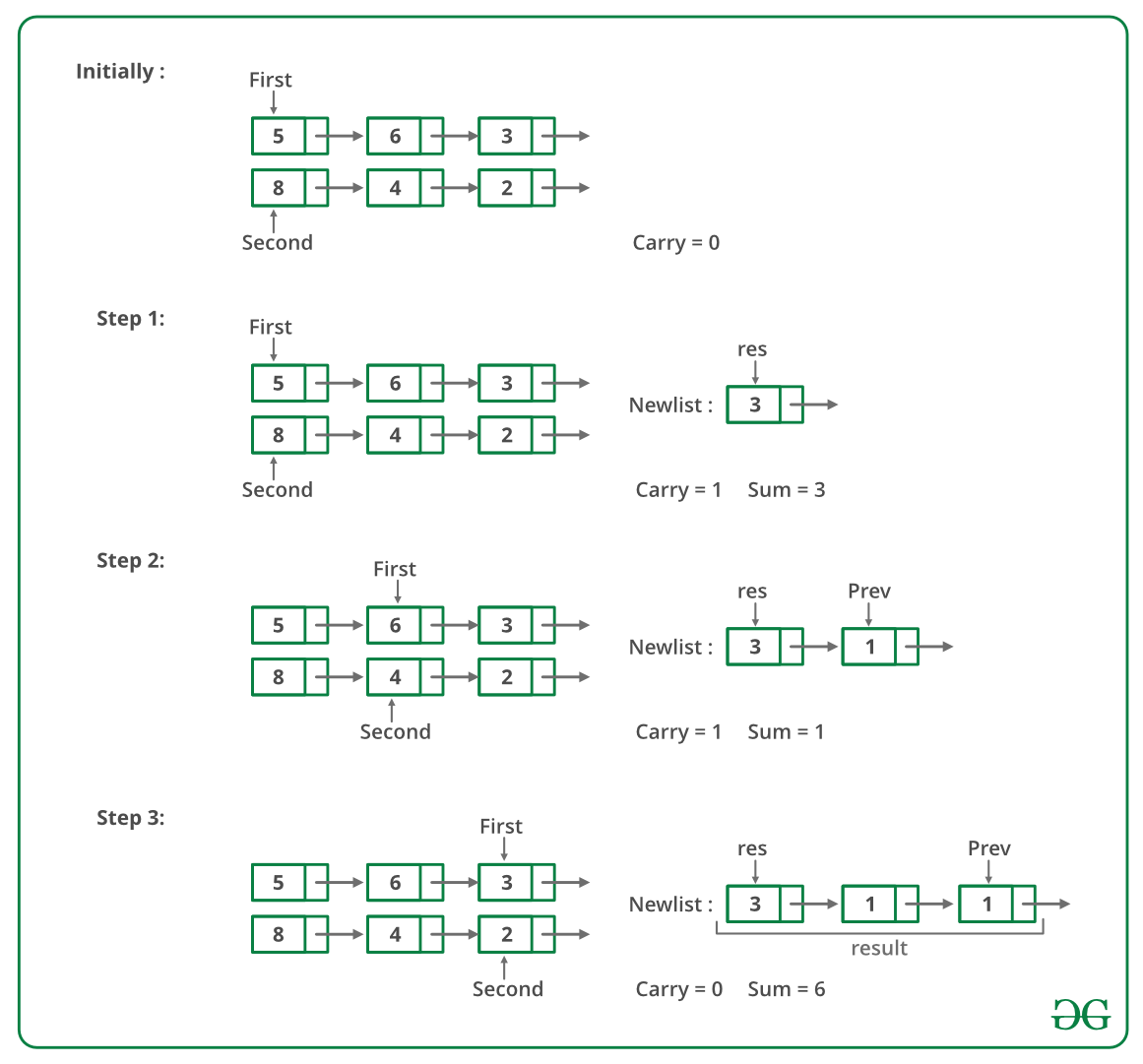GeeksforGeeks App
Open AppBrowser
Continue

# Javascript Program For Adding Two Numbers Represented By Linked Lists- Set 2

Given two numbers represented by two linked lists, write a function that returns the sum list. The sum list is linked list representation of the addition of two input numbers. It is not allowed to modify the lists. Also, not allowed to use explicit extra space (Hint: Use Recursion).

Example :

```Input:
First List: 5->6->3
Second List: 8->4->2

Output:
Resultant list: 1->4->0->5```

We have discussed a solution here which is for linked lists where a least significant digit is the first node of lists and the most significant digit is the last node. In this problem, the most significant node is the first node and the least significant digit is the last node and we are not allowed to modify the lists. Recursion is used here to calculate sum from right to left.

Following are the steps.

1. Calculate sizes of given two linked lists.
2. If sizes are same, then calculate sum using recursion. Hold all nodes in recursion call stack till the rightmost node, calculate the sum of rightmost nodes and forward carry to the left side.
3. If size is not same, then follow below steps:
• Calculate difference of sizes of two linked lists. Let the difference be diff.
• Move diff nodes ahead in the bigger linked list. Now use step 2 to calculate the sum of the smaller list and right sub-list (of the same size) of a larger list. Also, store the carry of this sum.
• Calculate the sum of the carry (calculated in the previous step) with the remaining left sub-list of a larger list. Nodes of this sum are added at the beginning of the sum list obtained the previous step.

Below is a dry run of the above approach:Below image is the implementation of the above approach.

## Javascript

 ``

Output:

`1 0 1 7`

Time Complexity: O(m + n) where m and n are the number of nodes in the given two linked lists.
Auxiliary Space:  O(m+n), where m and n are the number of nodes in the given two linked lists.

Iterative Approach:

This implementation does not have any recursion call overhead, which means it is an iterative solution.

Since we need to start adding numbers from the last of the two linked lists. So, here we will use the stack data structure to implement this.

• We will firstly make two stacks from the given two linked lists.
• Then, we will run a loop till both the stack become empty.
• in every iteration, we keep the track of the carry.
• In the end, if carry>0, that means we need extra node at the start of the resultant list to accommodate this carry.

## Javascript

 ``

Output:

`5 8 5`

Time Complexity: O(m + n) where m and n are the number of nodes in the given two linked lists.
Auxiliary Space:  O(m+n), where m and n are the number of nodes in the given two linked lists.

Related Article: Add two numbers represented by linked lists | Set 1 Please refer complete article on Add two numbers represented by linked lists | Set 2 for more details!

My Personal Notes arrow_drop_up